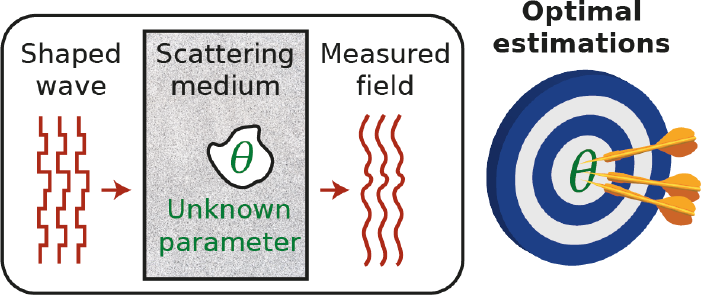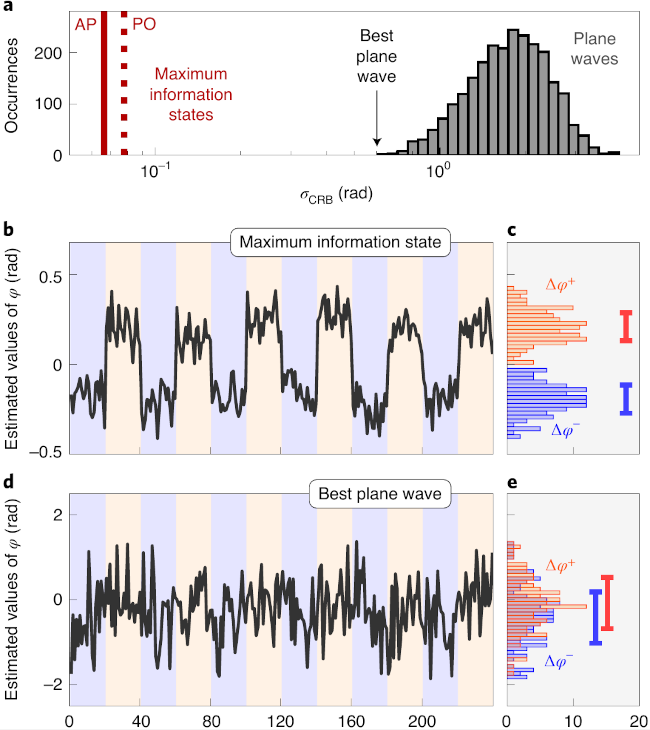## Maximum information states for coherent scattering measurements[D. Bouchet et al., Nat. Phys., 71 (2021)]

Coherent light is a popular tool for sensing and imaging. In simple cases, one can guess or compute a spatial and/or temporal excitation beam profile that ensures that the information about a specific target can be retrieved. However, there was no general rule to find the optimal states of light that maximize the precision of a given parameter estimation. Moreover, such states are expected to depend heavily on the parameter one tries to measure. In the present paper, the authors define a general framework to identify such optimal spatial channels, regardless of the complexity of the propagating medium, using the measurement of the transmission matrix. They demonstrate this concept using wavefront shaping to probe the phase and the position of a target hidden behind a static scattering medium.

#### Introduction

A parameter estimation based on physical measurements always comes with uncertainties imposed by the different sources of noise. It imposes a fundamental limit on the precision one can estimate physical observables, known as the Cramér-Rao bound. In wave physics, the Cramér-Rao bound can be approached by adapting the incoming wavefront. The amount of information retrieved from measurements about a given parameter for a specific experiment can be quantified by the Fisher information. In the present paper, the authors show that the wavefront that maximizes the Fisher information for the estimation of any physical parameter can be found using the knowledge of the transmission matrix of the system. The optimal states are given by the eigenstates of a Hermitian operator that quantifies the Fisher information. When one fully measures the response of the system, i.e. when one can measure the full scattering matrix of the optical system, the optimal solution found allows reaching the Cramér-Rao bound.

#### Principle

Let's consider that we want to estimate a parameter $$\theta$$. Our optical system is composed of a modulator illuminating a linear optical system, and a detector, that records the output field (in transmission, reflection, or both). A target parametrized by $$\theta$$ is hidden in or behind a complex linear system. In the paper, the experiment is done in reflection and the optical system consists in an optical diffuser with a target object hidden behind. The object is generated using a second spatial light modulator. The principle of the experiment is presented in Fig.1.

The reflection matrix $$r$$ of the system links the input field $$E^\text{in}$$ on the spatial light modulator to the output field $$E^\text{out}$$ on the camera:

$$\left| E^\text{out}\right\rangle= r\left| E^\text{in}\right\rangle \,\text{.}$$

The authors demonstrate that one can define an operator, called the Fisher information operator, defined as

$$f_\phi = (\partial_\theta r)^\dagger \partial_\theta r \,\text{.}$$

The eigenvector of this operator associated with the largest eigenvalue gives the incident state that maximizes the information received on the camera about the parameter to estimate, given the current state of the system and the measurement configuration. To proceed to the optimal estimation of this parameter, the phase mask corresponding to the optimal eigenstate is displayed on the modulator.

#### Experimental results

The authors show different experimental results to demonstrate this concept. In a first experiment, they choose as the parameter to estimate the global phase shift $$\theta =\Delta\phi$$ of a cross-shaped object hidden behind the diffuser as shown in Fig.2. The reflection matrix is measured for different values of $$\Delta\phi$$ and the Fisher information operator is estimated. The optimal eigenstate is computed and the corresponding phase mask is displayed on the SLM. We see in Fig.2 the intensity on the camera, as well as the amount of information carried by each of the camera pixels. They also show the sensitivity of changes in the object plane, i.e. roughly the amount of information that can be retrieved about each pixel of the generated object. We see that indeed, the optical mask allows being sensitive to changes only on the shape of the object. This is good, as it guarantees the best amount of information about the parameter to estimate, however, one can show that qualitatively similar results can be achieved by simply focusing on the target (e.g. using the transmission matrix or with a sequential optimization), which is typically easier to achieve.

To further demonstrate the advantage of the presented approach, the authors now restrict their measurements to a limited zone on the camera. The reflection matrix $$r$$ is then smaller. The optimal state still maximizes the sensitivity on the target by redirecting the intensity, and the information about the target, on the area of interest on the camera, as shown in Fig.3. This cannot be done by simply maximizing the optical intensity on the target.

Another experiment is presented in the paper to detect a displacement of an object.

Finally, the authors compare the precision limit, which is related to the amount of noise in the reconstructed signal, in the case of the optimal state and the different plane waves that were sent to reconstruct the reflection matrix $$r$$. They observe one order of magnitude difference compared to the best plane wave (see Fig.4a.). While other approaches allow finding solutions already better than a plane wave, this technique ensures optimal results. In Fig.4 b and c are shown the reconstruction of small periodic oscillation of the phase of the target. The optimal state allows a clear reconstruction of the signal while the plane wave input beam gives rise to seemingly random fluctuations.Figure 4.  a, Precision limit histogram for the different plane waves as input wavefront compared to the optimal state (AP) and the one with only phase modulation (PO), which is what is achievable using a liquid crystal phase modulator. b,c, estimation of the phase in the case of the optimal state and with a plane wave.